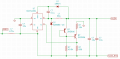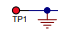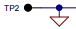# Negative buck converter, does this work?

#### Veracohr

Joined Jan 3, 2011
765
I have a need for regulated +/-15V from an unregulated bipolar supply that may range from 25-35V under varying load conditions. I want to use a buck converter so I don't have to heat sink anything. Efficiency is not really a concern for this. For the positive side I plan to use MCP16331 and it seems straightforward.

For the negative side I had a hard time finding examples that took a negative voltage and stepped it "down" to a negative voltage of lower absolute value. Mostly there are examples of a positive-input, negative-output buck-boost configuration. However I found this application note for a switching controller with a similar idea but somewhat more complicated. What I'm taking from that application note is the method of referencing the feedback voltage to the input supply common rather than the controller's "GND" pin, which in this case is actually the input voltage.

The feedback pin of the MCP16331 should be 0.8V above the GND pin when in regulation, so the idea here is to use a current source to keep the feedback pin 0.8V above the "N15V_RTN" net, which is the same as the input VNEG net at DC, when the output voltage is -15V. That way VNEG can vary from -25V to -35V but the regulator should keep the output at -15V since it's referenced to the supply common rather than the converter's own GND pin.

See any glaring problems with this?#### crutschow

Joined Mar 14, 2008
31,146
Unfortunately one thing does glare at me.The negative common is not the same at the input and output because the voltage is regulated by switching the ground side of the voltage.
Thus both outputs must be isolated from the input ground.
That won't work if the positive ground needs to be the same as the negative ground.
I assume your unregulated plus and minus supply outputs share a ground.

This circuit would work better if that's the case, as it switches the negative voltage so the negative ground is common between the input and output.
Thus the plus and minus outputs can share the ground.

The schematic is a little confusing, since it appears to show two different ground symbols.

Thisis the circuit common (ground).

Thisis the negative input which goes to wherever else in the circuit you see that symbol.

TP4 is the regulated negative output.

Last edited:

#### Veracohr

Joined Jan 3, 2011
765

#### crutschow

Joined Mar 14, 2008
31,146
Does this look better?
Not really.
Okay, looking at your original circuit I see an error in the pin identification which is causing confusion.
The two output labels are transposed.
The one labeled N15V is really N15V_RTN and the one labeled N15V_RTN is really N15V.
So the VNEG line is the N15V output (with respect to N15V_RTN).

And since it is the common line that is being switched to regulate the voltage, the N15V_RTN can't be connected to circuit ground.

So the actual major difference between the two is the line being switched to regulate the voltage.
You need a regulator where the switch is in the VNEG lead such as the one I posted.

Make sense?

Edit: I just noticed the design in the app note you referenced has the switch in the negative line, so can also work with a common ground.
A controller IC with a internal MOSFET switch, such as the 16331, likely will not work for you, since they are designed to switch the most positive rail, not the most negative.

Last edited: